Computational Complexity and the Anthropic Principle

Notes for a talk given at the Stanford Institute for Theoretical Physics, December 15, 2006

When Lenny [Susskind] and Alessandro [Tomasiello] invited me to speak here, I said, "I'll be delighted to, as long you realize that my understanding of string theory is about at the level of Brian Greene's TV show."

There's a relevant joke -- I don't know if you've heard it. A mathematician and an engineer are sitting in on a string theory lecture. The engineer is struggling, while the mathematician is swimming along with no problem. Finally the engineer asks, "How do you do it? How do you visualize these 11-dimensional spaces?" The mathematician says, "It's easy: first I visualize an n-dimensional space, then I set n equal to 11."

My background is in theoretical computer science. In this field we don't know anything about 11-dimensional spaces, but we do know something about n-dimensional spaces. So when string theorists talk (as you do now) about a landscape of exponentially many vacua, in some sense you've blundered onto our territory. I guess the first thing I should say is, welcome!

What I want to do today is step back from the details of the string-theory Landscape, and tell you what's known about the general problem of searching a landscape for a point with specified properties. In particular, is this problem solvable in a reasonable amount of time using the resources of the physical universe? And if, as many of us think, the answer is no, then what are the implications for physics?

Complexity 101

I'll start with a crash course in computational complexity theory -- in the basic concepts that we'll need even to talk about these issues.

In computer science, we like to restrict attention to questions that are specified by a finite sequence of bits, and that have a definite yes-or-no answer. So for example: "Given a graph, is it connected?" (I.e., is every vertex reachable from every other vertex?)

But crucially, if you I give you a graph and ask if it's connected, I haven't yet given you a problem. I've merely given you an instance. The set of all possible graphs -- now that's a problem! The reason we talk this way is that we want a general-purpose algorithm -- one that will take as input any possible graph, and determine in a reasonable amount of time whether it's connected.

Alright, what do we mean by a "reasonable amount of time"? We mean that the algorithm should output the right answer after at most cnk steps, where n is the "problem size" (usually, just the number of bits needed to write down the problem instance), and c and k are constants independent of n. If this holds, then we say the algorithm is polynomial-time.

No sooner do you state this definition than people start coming up with objections: what if an algorithm took n10000 steps? Surely we wouldn't want to call that efficient! Conversely, what if it took 1.00000001n steps? Surely that's efficient for all practical purposes!

Two responses:

1. Empirically, most problems really do seem to sort themselves into (a) polynomial-time ones that (while often difficult) are not completely hopeless, and (b) exponential-time ones that are completely hopeless. It could've turned out otherwise.
2. Even more important, identifying polynomial-time with efficient is convenient; it lets us get on with building a theory. Many of the qualitative insights that we get from this theory are extremely general, and would continue to apply even if we replaced polynomial-time with some other criterion for efficiency.

Alright, the next step is to consider the class of all problems that are solvable by polynomial-time algorithms. This class we call P (for Polynomial-Time). What are some examples of problems in P?

• The example I mentioned before: given a graph, decide if it's connected.
• A highly nontrivial example: given a positive integer, decide if it's prime. (Only known as of four years ago!)
• The linear programming problem: given a set of linear inequalities, decide if they can be satisfied simultaneously.

But other problems are not known to be in P:

• Given a map, can every country be colored red, green, or blue so that no neighboring countries are colored the same?
• Given a collection of boxes, can they be packed in your trunk?
• Given a collection of cities, together with the distances between them, is there a route of at most 10,000 miles that visits every city once?
• Given a Boolean formula, is there a setting of the variables that makes it true?

However, the above problems belong to a bigger class called NP, for Nondeterministic Polynomial-Time. A problem is in NP if, whenever the answer is "yes," there's a polynomial-size proof of that fact that can be checked in polynomial time. Not all problems are in NP: for example, given a Turing machine, will it ever halt?

(Why is it called "nondeterministic"? Because we imagine a machine that could "nondeterministically guess" the right answer. But don't worry about this -- it was a bad choice of name to begin with.)

We say a problem is NP-hard if any NP problem can be efficiently reduced to it -- in other words, if there's a polynomial-time algorithm that translates any instance of an NP problem into an instance of the problem in question. Then a problem is called NP-complete if it's both NP-hard and in NP. In other words, NP-complete problems are "the hardest problems in NP." The big discovery that really got the field started, in the early seventies, is that not just one or two but thousands of natural search problems are NP-complete. So for example, the map-coloring, box-packing, Traveling Salesman, and Boolean satisfiability problems are all NP-complete. This means that an efficient solution to any one of them would imply efficient solutions to all the rest: if you find a way to color maps, then you've also found a way to pack boxes in your trunk, and so on. In a very precise sense, all these problems are just different encodings of the same thing.The (literally) million-dollar question is of course if P=NP. Equivalently, can any NP-complete problem be solved in polynomial time? We all believe that PNP. Why? Partly because of the failure, over half a century, to find any algorithms for NP-complete problems that are fundamentally better than brute-force search. We do have local search algorithms that sometimes do extremely well in practice, but as you know, there's no guarantee that such methods won't get stuck at local optima. But another reason we believe PNP is that otherwise mathematical creativity could be automated! You'd simply ask your computer: "Is there a proof of the Riemann hypothesis with at most a million symbols?" And if there is such a proof, the computer will find it in some way dramatically faster than searching through every possibility. If P=NP, you wouldn't merely have solved one of the seven million-dollar problems from the Clay Math Institute -- presumably you could also solve the other six.

If the computers we have today can't solve NP-complete problems in polynomial time, then what about quantum computers? As most of you know, in 1994, there was this spectacular discovery that quantum computers can factor integers in polynomial time, and thereby break the RSA cryptosystem. But contrary to a popular misconception, the factoring problem is not believed to be NP-complete. It's certainly in NP, and we don't believe it's in P (that belief is what the RSA cryptosystem is based on), but it seems intermediate between P and NP-complete. One way to understand the difference between factoring and the known NP-complete problems is this. If I give you a collection of boxes, there might be zero ways to pack them into your trunk, or 10 ways, or 40 billion ways. But if I give you a positive integer, you know in advance that it has exactly one prime factorization -- even if you don't know what it is! (What makes the factoring problem special -- and what Peter Shor exploited in his algorithm -- goes much deeper than this uniqueness property; it has to do with the fact that factoring is reducible to another problem called period-finding. Uniqueness is just the first hint that we're not dealing with a "generic" search problem.)

So the question remains: can quantum computers solve the NP-complete problems in polynomial time? Well, today we have strong evidence that the answer is no. Of course we can't prove that there's no fast quantum algorithm -- we can't even prove that there's no fast classical algorithm! But what we can say is this. Suppose you threw away the structure of an NP-complete problem, and just considered a space (or landscape) of 2n possible solutions, together with a "black box" that tells you whether a given solution is correct or not. Then how many steps would it take to find a solution, supposing you could query the black box in quantum superposition? The answer turns out to be about 2n/2. So, you can do quadratically better than a classical algorithm -- but only quadratically better. If n is (say) 100,000, then instead of 2100,000 steps, you now need "merely" 250,000 steps. Whup-de-doo!

Even if we do take the structure of NP-complete problems into account when designing quantum algorithms, the outlook is still pretty bleak. We do have a quantum analog of local search, called the quantum adiabatic algorithm. And in some situations where a classical local search algorithm would get trapped at a local minimum, the adiabatic algorithm is able to "tunnel" through potential barriers and thereby reach a global minimum. But only in some situations! In other situations, the adiabatic algorithm does almost as poorly as classical local search.

These findings suggest a more general question: is there any physical means to solve NP-complete problems in polynomial time? Maybe by going beyond quantum computers and exploiting closed timelike curves, or hypothetical nonlinearities in the Schr�dinger equation, or quantum gravity effects that only become important at the Planck scale or near a black hole singularity? Well, it's good to keep an open mind about these things. But my own opinion is that eventually, the hardness of NP-complete problems will be seen the same way as (say) the Second Law of Thermodynamics or the impossibility of superluminal signalling are seen today. In other words: these are overarching meta-principles that are satisfied by every physical theory we know. Any of them might be falsified tomorrow, but it seems manifestly more useful to assume they're true, and then see what the consequences are for other issues! (Cf. A. Einstein, J. Bekenstein.)

Alright, let me put my chalk where my mouth is:

The NP Hardness Assumption: There is no physical means to solve NP-complete problems in polynomial time.

There are many criticisms you could make of this assumption. But one criticism I don't think you can make is that it's outside the scope of science. This assumption is about as operational as it gets! It refers directly to experience, to what sorts of computations you could actually perform. It's obvious what it would take to falsify it. The only thing you might legitimately complain about is the reliance on asymptotics -- but if that's your beef, then just substitute any reasonable explicit numbers you want!

Complexity and the Landscape

The reason I'm here is that a year ago, Frederik Denef and Mike Douglas wrote a very interesting paper called "Computational complexity of the landscape." In this paper they considered toy problems of the following sort: we're given a collection of N scalar fields φ1,...,φn, each of which could make one of two possible contributions to the vacuum energy Λ, depending on the choice of moduli. How do we choose the moduli so as to minimize |Λ|? Denef and Douglas proved that such problems are NP-hard, by reduction from the Subset Sum problem. From a computer science perspective, this is not at all a surprising result -- it's exactly what one would have expected. Even so, I find it gratifying that string theorists are now asking this sort of question.

There's a crucial caveat to the Denef-Douglas paper -- a sort of asterisk hanging over every sentence -- and it's one that Denef and Douglas themselves are perfectly well aware of. This is that, as physicists, you're not interested in arbitrary collections of scalar fields. You're interested in some particular set of fields -- for example, the ones found in Nature! And computational complexity theory never says anything directly about the hardness of individual instances. One can never rule out the possibility that there's some structure, some symmetry, some insight, that would let you solve the particular instance you care about without solving the general case.

The Anthropic Principle

That's really all I wanted to say about the nitty-gritty practical question of finding a Calabi-Yau manifold that reproduces the Standard Model. But since you're a captive audience, I thought I'd now take the liberty of pontificating about the Anthropic Principle. I've heard vague rumors that there's been a bit of controversy about this lately -- is that true?

Lenny Susskind: If by "controversy" you mean any serious alternatives, then no, there hasn't been...
Scott: In other words, yes, there has...

Usually the question is framed as, "Is the Anthropic Principle a legitimate part of science?" But that's the wrong question. I hope to convince you that there are cases where we'd all be willing to apply the Anthropic Principle, and other cases where none of us would be willing to apply it -- presumably not even Lenny! [Lenny's response: "Try me!"]

To start with one of the "good" examples: why is our planet 93 million miles from the sun? Presumably we all agree that, to the extent this question has an answer at all, it's the usual Goldilocks answer (not too hot, not too cold, just right). But now consider some other examples:

1. Why do I not have red hair? Well, if I did, then clearly I wouldn't be asking why I didn't. So the very fact that I'm asking the question means my hair can't be red.
2. Why is the moon round? Because one night my parents were walking on the beach, entranced by the beauty of the round moon. And that's the night I was conceived. If the moon were square, I wouldn't exist to ask the question.
3. Suppose there are two competing cosmological models. One model leads to a finite universe, the other leads to an infinite universe. Cosmologists are about to launch a space probe that will test which model is correct. But then philosophers come along and say, "Wait -- you don't have to bother! Obviously the infinite model must be the correct one -- since in an infinite universe, we'd be infinitely more likely to exist in the first place!" The question I'd put to anthropicists is this: should the philosophers win the Nobel Prize that would have otherwise gone to the cosmologists? And if not, why not? (This example is due to the philosopher Nick Bostrom.)

I hope I've convinced you that there are cases where all of us would be willing to use the Anthropic Principle, and also cases where none of us would. If you accept this, then we can immediately throw away the question of whether the anthropic principle is a "valid part of science." We can instead ask a better question: in what situations is it valid? And this is where I'll argue that the NP Hardness Assumption can contribute something.

But what could NP-hardness possibly have to do with the Anthropic Principle? Well, when I talked before about computational complexity, I forgot to tell you that there's at least one foolproof way to solve NP-complete problems in polynomial time. The method is this: first guess a solution at random, say by measuring electron spins. Then, if the solution is wrong, kill yourself! If you accept the many-worlds interpretation of quantum mechanics, then there's certainly some branch of the wavefunction where you guessed right, and that's the only branch where you're around to ask whether you guessed right! It's a wonder more people don't try this.

One drawback of this algorithm is that, if the problem instance didn't have a solution, then you'd seem to be out of luck! But there's a simple way to fix this: with tiny probability, don't do anything. Then, if you find yourself in the branch where you didn't do anything, you can infer that there almost certainly wasn't any solution -- since if there was a solution, then you probably would've found yourself in one of the universes where you guessed one.

There's a less gruesome way to get a similar result. Let's suppose that in the far future, it's possible to clone yourself. I don't mean a genetic twin -- I mean someone whose memories, neural patterns, etc. are completely identical to yours. "Clearly," you should be more likely to exist in a branch of the wavefunction where there are millions of such beings running around than in a universe where there's only one (since for all you know, you could be any one of the clones!). And this leads immediately to another "algorithm" for NP-complete problems: First guess a random solution. If the solution is wrong, don't do anything. If the solution is right, then bring exponentially many clones of yourself into existence.

Now, you might think that creating exponentially many clones of yourself would itself require exponential time. But in a de Sitter universe, like the one we seem to inhabit, that simply isn't true. For you can simply make two clones of yourself, then have those clones travel (say) 10 billion light-years away from each other, then have both of them make two more clones, who then travel 10 billion light-years away from each other, and so on (say) 2n times in a geometric progression. Since the cosmological constant Λ is positive, the scale factor of the universe is increasing exponentially the whole time, so the clones will never run out of room to expand. There's no problem of principle here.

Alright, so suppose there are 2n possible solutions. In a branch of the wavefunction where you guessed a correct solution, you're 22n times as likely to exist in the first place as in a universe where you didn't. Therefore, you're overwhelmingly likely to find yourself in a universe where you guessed a correct solution.

Now, if you accept the NP Hardness Assumption (as I do), then you also believe that whatever else might be true of the Anthropic Principle, the previous two examples must have been incorrect uses of it. In other words, you now have a nontrivial constraint on anthropic theorizing:

No application of the Anthropic Principle can be valid, if its validity would give us a means to solve NP-complete problems in polynomial time.

Incidentally, this constraint leads to the strongest argument against suicide I know of. Namely, if suicide really were a solution to life's problems, then one could exploit that fact to solve NP-complete problems in polynomial time! I'm thinking of starting a suicide hotline for computer scientists, where I'll explain this point to them.

Anthropic Quantum Computing

I feel like I should give you at least one nontrivial result -- so you can come away from the talk feeling like you've gotten your recommended daily allowance of math, and I can convince you that I actually do something for a living.

Alright, so let's say you combine quantum computing with the Anthropic Principle. In other words, let's say you do postselection not on the outcome of a classical computation, but on the outcome of a quantum computation. That is, you perform an ordinary polynomial-time quantum computation, except that you now have the ability to make a measurement, kill yourself if the measurement doesn't yield a desired outcome, and then consider only those branches of the wavefunction in which you remain alive.

The class of problems solvable efficiently on an ordinary quantum computer is called BQP: Bounded-Error Quantum Polynomial-Time. When we throw in postselection, we immediately get a bigger complexity class, which we can give a name: PostBQP, or Postselected BQP. But exactly how big is this class?

Well, we saw before that even classical postselection would let us solve NP-complete problems in polynomial time. So certainly PostBQP contains NP. But would quantum postselection let us do even more than NP-complete problems?

To address that question, I need to introduce another animal, called PP. PP -- yeah, I know, it's a stupid name -- stands for "Probabilistic Polynomial-Time." Informally, PP is the class of problems of the form, "Of the 2n possible solutions to this problem, are at least half of them correct?" In other words, PP is a generalization of NP where instead of just deciding whether a solution exists, we now want to count the number of solutions.

A beautiful result from ten years ago, due to Adleman, DeMarrais, and Huang, says that BQP, the class of problems solvable efficiently on a quantum computer, is contained in PP. The reason why this is so can be explained to a physicist in three words: "Feynman path integral." The probability that a quantum computer outputs "yes" can be expressed as an exponentially-large sum over all possible paths -- some of which contribute positive amplitude to the final answer, others of which contribute negative amplitude. But an exponentially-large sum is exactly the sort of thing that can be calculated in PP. From my perspective, Richard Feynman won the Nobel Prize in physics essentially for showing that BQP is contained in PP.

The proof that BQP is contained in PP easily extends to show that PostBQP is contained in PP: you now just restrict the sum to the paths that are going to be postselected.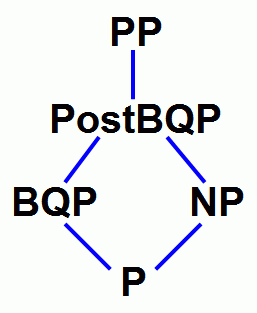Is the converse also true? In other words, is PP contained in PostBQP? A couple years ago, I managed to show that the answer is yes. Hence PostBQP=PP: this weird quantum complexity class is exactly equivalent to a well-studied classical class.

Let me sketch for you how the proof goes. We have a Boolean function f:{0,1}n-->{0,1}, which maps an n-bit input to a 1-bit output. Let s be the number of inputs x such that f(x)=1. Then our goal is to decide whether s≥2n-1. Assume for simplicity (and without loss of generality) that s>0.

Using standard quantum computing tricks (which I'll skip), it's relatively easy to prepare a one-qubit state of the form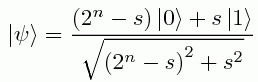.

So for some real numbers α,β to be specified later, we can also prepare the state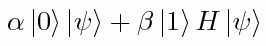,

where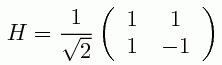is the so-called Hadamard transformation, andis the result of applying that transformation to |ψ>.

Now let's postselect on the second qubit being |1>. Then the reduced state of the first qubit will be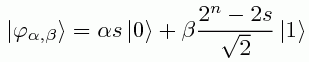divided by a suitable normalizing factor.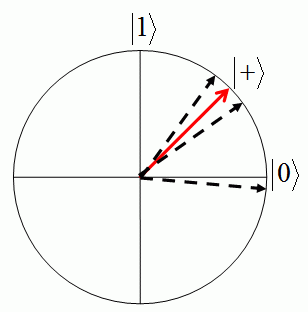Alright, now suppose we vary the ratio β/α through 2-n, 2-(n-1), ..., 1/2, 1, 2, ..., 2n-1, 2n, but always keeping α and β positive. Then there are two possible cases, as shown in the above diagram:

1. If s<2n-1, then the amplitudes αs andwill both be positive. So as we vary α and β, eventually the state |φα,β> will get reasonably close to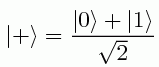.

2. If s≥2n-1, then αs will be positive butwill not be positive. So |φα,β> will never even get into the first quadrant, and hence will always be far from |+>.

The two cases above can be efficiently distinguished from each other! For each value of β/α, just prepare (say) O(log n) copies of |φα,β>, measure each one in the {|+>,|->} basis, and count how many times you get |+>. This completes the proof that PP is contained in PostBQP.

OK, so quantum anthropic computing has exactly the power of PP. This seems like a fun result, but not the sort of thing you'd want to base your tenure case on! But a year after proving it, I realized something odd. For 20 years, a famous open problem was to prove that PP is closed under intersection: in other words, if you have two PP algorithms A and B, then there's a third PP algorithm that answers yes on a given input if and only if A and B both do. This problem was finally solved by Beigel, Reingold, and Spielman in 1991, using sophisticated techniques involving "low-degree threshold polynomials." But notice that PostBQP is trivially closed under intersection. If you have two PostBQP problems, then you can simply run the algorithms for both of them, postselect on both of them succeeding, and then accept if and only if they both do. Therefore, my result that PostBQP=PP immediately yields a half-page proof that PP is closed under intersection.

So thinking about the Anthropic Principle turned out to be good for something after all! And that leads to my concluding thought. When scientists attack the Anthropic Principle -- say, in the context of the string landscape -- it's possible that some of them really are "Popperazzi," trying to enforce a preconceived notion of what and isn't science. But for many of them, I think the unease comes from a different source. Namely, suppose we do invoke the Anthropic Principle. Is this scientifically fruitful? Does it lead to any nontrivial results? If this talk has an optimistic message, it's that puzzling over the Anthropic Principle has certainly been fruitful for me! It's led me to computer science results that I wouldn't have thought of otherwise. Of course, if it hadn't, then presumably I wouldn't be speaking here in the first place, so there might be an anthropic component to that as well.